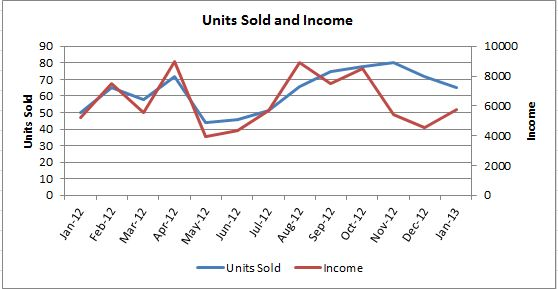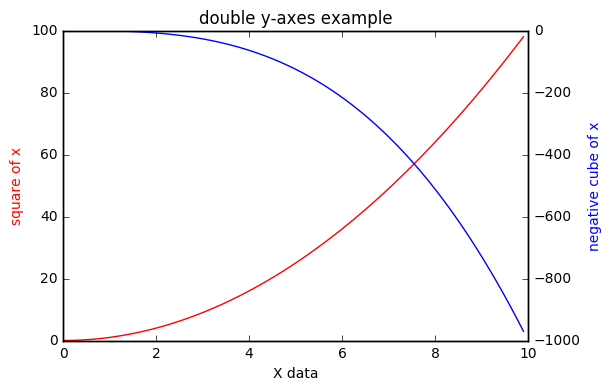#### python怎么画excel里那种双y轴的图？

统计/机器学习 数据可视化 Python    浏览次数：7093        分享

0

python怎么画excel里那种双y轴的图？类似这样的，左边和右边各一个纵轴LeBron   2017-05-12 09:49

##### 1个回答
4

import numpy as np

import matplotlib.pyplot as plt

x = np.arange(0, 10, 0.1)

y1 = x ** 2

y2 = - x ** 3

fig, ax1 = plt.subplots()

ax2 = ax1.twinx()

ax1.plot(x, y1, 'r-')

ax2.plot(x, y2, 'b-')

ax1.set_xlabel('X data')

ax1.set_ylabel('square of x', color='r')

ax2.set_ylabel('negative cube of x', color='b')

plt.title('double y-axes example')

plt.show()SofaSofa数据科学社区DS面试题库 DS面经五道口少年   2017-05-12 11:34相关主题